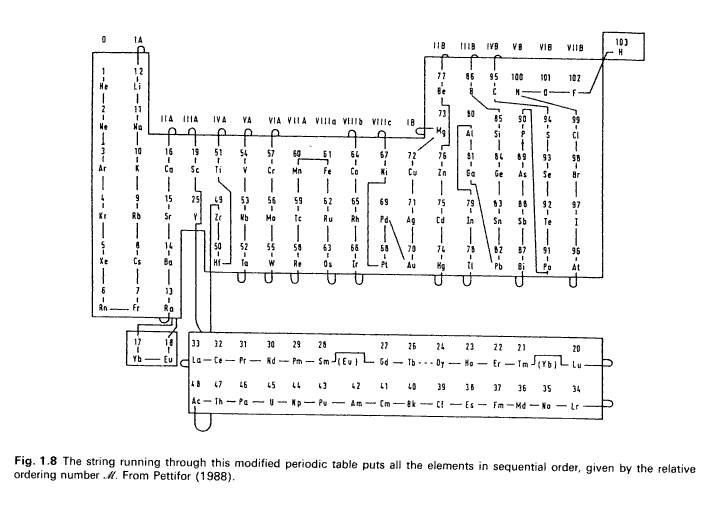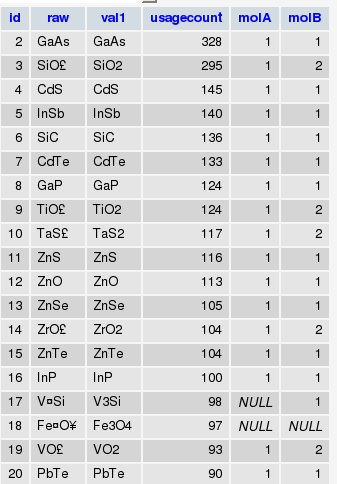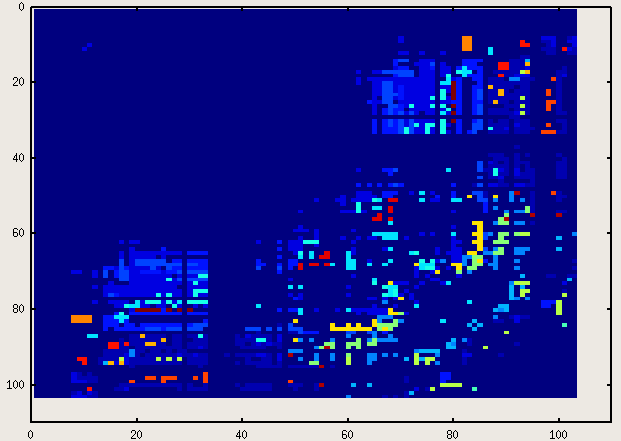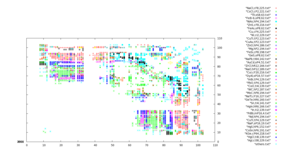# Hex, Bugs and More Physics | Emre S. Tasci

a blog about physics, computation, computational physics and materials…

## Element Arrays

### April 10, 2008 Posted by Emre S. Tasci

For future reference when coding, I thought it would be useful to include these arrays. All of the arrays are ordered with respect to their Atomic Numbers.

Atomic Numbers Array

"1", "2", "3", "4", "5", "6", "7", "8", "9", "10", "11", "12", "13", "14", "15", "16", "17", "18", "19", "20", "21", "22", "23", "24", "25", "26", "27", "28", "29", "30", "31", "32", "33", "34", "35", "36", "37", "38", "39", "40", "41", "42", "43", "44", "45", "46", "47", "48", "49", "50", "51", "52", "53", "54", "55", "56", "57", "58", "59", "60", "61", "62", "63", "64", "65", "66", "67", "68", "69", "70", "71", "72", "73", "74", "75", "76", "77", "78", "79", "80", "81", "82", "83", "84", "85", "86", "87", "88", "89", "90", "91", "92", "93", "94", "95", "96", "97", "98", "99", "100", "101", "102", "103", "104", "105", "106", "107", "108", "109", "110", "111", "112", "113", "114", "115", "116", "117", "118"

Symbols Array

"H", "He", "Li", "Be", "B", "C", "N", "O", "F", "Ne", "Na", "Mg", "Al", "Si", "P", "S", "Cl", "Ar", "K", "Ca", "Sc", "Ti", "V", "Cr", "Mn", "Fe", "Co", "Ni", "Cu", "Zn", "Ga", "Ge", "As", "Se", "Br", "Kr", "Rb", "Sr", "Y", "Zr", "Nb", "Mo", "Tc", "Ru", "Rh", "Pd", "Ag", "Cd", "In", "Sn", "Sb", "Te", "I", "Xe", "Cs", "Ba", "La", "Ce", "Pr", "Nd", "Pm", "Sm", "Eu", "Gd", "Tb", "Dy", "Ho", "Er", "Tm", "Yb", "Lu", "Hf", "Ta", "W", "Re", "Os", "Ir", "Pt", "Au", "Hg", "Tl", "Pb", "Bi", "Po", "At", "Rn", "Fr", "Ra", "Ac", "Th", "Pa", "U", "Np", "Pu", "Am", "Cm", "Bk", "Cf", "Es", "Fm", "Md", "No", "Lr", "Rf", "Db", "Sg", "Bh", "Hs", "Mt", "Ds", "Rg", "Uub", "Uut", "Uuq", "Uup", "Uuh", "Uus", "Uuo"

Names Array

"Hydrogen", "Helium", "Lithium", "Beryllium", "Boron", "Carbon", "Nitrogen", "Oxygen", "Fluorine", "Neon", "Sodium", "Magnesium", "Aluminum", "Silicon", "Phosphorus", "Sulfur", "Chlorine", "Argon", "Potassium", "Calcium", "Scandium", "Titanium", "Vanadium", "Chromium", "Manganese", "Iron", "Cobalt", "Nickel", "Copper", "Zinc", "Gallium", "Germanium", "Arsenic", "Selenium", "Bromine", "Krypton", "Rubidium", "Strontium", "Yttrium", "Zirconium", "Niobium", "Molybdenum", "Technetium", "Ruthenium", "Rhodium", "Palladium", "Silver", "Cadmium", "Indium", "Tin", "Antimony", "Tellurium", "Iodine", "Xenon", "Cesium", "Barium", "Lanthanum", "Cerium", "Praseodymium", "Neodymium", "Promethium", "Samarium", "Europium", "Gadolinium", "Terbium", "Dysprosium", "Holmium", "Erbium", "Thulium", "Ytterbium", "Lutetium", "Hafnium", "Tantalum", "Tungsten", "Rhenium", "Osmium", "Iridium", "Platinum", "Gold", "Mercury", "Thallium", "Lead", "Bismuth", "Polonium", "Astatine", "Radon", "Francium", "Radium", "Actinium", "Thorium", "Protactinium", "Uranium", "Neptunium", "Plutonium", "Americium", "Curium", "Berkelium", "Californium", "Einsteinium", "Fermium", "Mendelevium", "Nobelium", "Lawrencium", "Rutherfordium", "Dubnium", "Seaborgium", "Bohrium", "Hassium", "Meitnerium", "Darmstadtium", "Roentgenium", "Ununbium", "Ununtrium", "Ununquadium", "Ununpentium", "Ununhexium", "Ununseptium", "Ununoctium"

Pettifor’s Mendeleev Numbers Array
D.G. Pettifor, Mater. Sci. Tech. 4 (1988) 2480 | D.G. Pettifor, Bonding and Structure of Molecules and Solids, Oxford University Press (1995) p.13"103", "1", "12", "77", "86", "95", "100", "101", "102", "2", "11", "73", "80", "85", "90", "94", "99", "3", "10", "16", "19", "51", "54", "57", "60", "61", "64", "67", "72", "76", "81", "84", "89", "93", "98", "4", "9", "15", "25", "49", "53", "56", "59", "62", "65", "69", "71", "75", "79", "83", "88", "92", "97", "5", "8", "14", "33", "32", "31", "30", "29", "28", "18", "27", "26", "24", "23", "22", "21", "17", "20", "50", "52", "55", "58", "63", "66", "68", "70", "74", "78", "82", "87", "91", "96", "6", "7", "13", "48", "47", "46", "45", "44", "43", "42", "41", "40", "39", "38", "37", "36", "35", "34", "0", "0", "0", "0", "0", "0", "0", "0", "0", "0", "0", "0", "0", "0", "0"

Villars’ Mendeleev Numbers Array
P. Villars et al., J. Alloys Compd. 367 (2004) 167] –doi:10.1016/j.jallcom.2003.08.060"92", "98", "1", "67", "72", "77", "82", "87", "93", "99", "2", "68", "73", "78", "83", "88", "94", "100", "3", "7", "11", "43", "46", "49", "52", "55", "58", "61", "64", "69", "74", "79", "84", "89", "95", "101", "4", "8", "12", "44", "47", "50", "53", "56", "59", "62", "65", "70", "75", "80", "85", "90", "96", "102", "5", "9", "13", "15", "17", "19", "21", "23", "25", "27", "29", "31", "33", "35", "37", "39", "41", "45", "48", "51", "54", "57", "60", "63", "66", "71", "76", "81", "86", "91", "97", "103", "6", "10", "14", "16", "18", "20", "22", "24", "26", "28", "30", "32", "34", "36", "38", "40", "42", "0", "0", "0", "0", "0", "0", "0", "0", "0", "0", "0", "0", "0", "0", "0"

You can also download the SQL version for these values from here.

Hope it helps..

## Pettifor Map 2000 Edition

### April 9, 2008 Posted by Emre S. Tasci

Suppose you have the formulas, structures and temperature and pressure of the measuring environment stored in your database. Here are some steps to draw a 2000 version of the Pettifor Map.

First of all, here is what my formula table in the database looks like:The information in molA and molB columns are populated from formulas using the following MySQL commands:

AB
UPDATE dbl014 SET molA=1, molB=1 WHERE val1 NOT REGEXP "[0-9]"

AB[0-9]
UPDATE `dbl014` SET molA=1 WHERE val1 NOT REGEXP "[0-9][A-Z]" AND molA IS NULL

A[0-9]B
UPDATE `dbl014` SET molB=1 WHERE val1 NOT REGEXP "[0-9]\$" AND molB IS NULL

A2B
UPDATE `dbl014` SET molA=2 WHERE val1 REGEXP "[A-z]2[A-z]" AND molB =1

AB2
UPDATE `dbl014` SET molB=2 WHERE val1 REGEXP "[A-z]2\$" AND molA =1

But we will be focusing only to the AB compositon today.

In addition, I have another table where I store information about elements:So I can go between Symbol to Atomic Number to Mendeleev Number as I please..

Here is what I did:

* Retrieved the complete list of AB compositions.

* Retrieved the reported structures and the temperature and pressure of the entries for each composition.

* Filtered the ones that weren’t measured/calculated at ambient pressure

* Also recorded each component’s Mendeleev number, so at the end I obtained the following table where formula and structure information is enumerated (for instance, the values you see in the formula column are nothing but the ids of the formulas you can see in the first figure):* Next, selected those that were measured in ambient temperature (something like SELECT * FROM db.table GROUP BY structure, formula WHERE temp=298)

* Builded a histogram for structure populations, took the first 10 most populated structures as distinct and joining the rest as "other" (assigned structure number of "0"). By the way, here are the top 20 structures for AB compositions with the first number in parentheses showing its rank among binary compounds while the second is the number AB compositions registered in that structure type:

1 — NaCl,cF8,225 (4) (347)
2 — CsCl,cP2,221 (7) (306)
3 — TlI,oS8,63 (14) (126)
4 — FeB-b,oP8,62 (32) (69)
5 — NiAs,hP4,194 (16) (62)
6 — ZnS,cF8,216 (9) (51)
7 — CuAu,tP2,123 (44) (42)
8 — CuTi,tP2,123 (76) (34)
9 — Cu,cF4,225 (3) (34)
10 — FeAs,oP8,62 (51) (30)
11 — ZnO,hP4,186 (23) (28)
12 — W,cI2,229 (2) (26)
13 — FeSi,cP8,198 (57) (25)
14 — Mg,hP2,194 (5) (25)
15 — NaPb,tI64,142 (207) (14)
16 — ZrCl,hR12,166 (365) (14)
17 — NaO,hP12,189 (208) (13)
18 — AuCd,oP4,51 (121) (10)
19 — WC,hP2,187 (138) (10)
20 — DyAl,oP16,57 (149) (10)

* Wrote these data into a file ("str_wo_temp.txt" for further references) with [a] | [b] | [structure] being the columns as in :
79  100 11
26  93  11
24  93  11
73  92  11
78  100 11
27  64  0
66  92  0
12  97  0
53  101 0
61  86  4
31  84  4
23  85  4
32  85  4
33  85  4
27  85  4

so it was piece of a cake (not that easy, actually 8) to plot this using gnuplot :

set term wxt
set palette rgb 33,13,10
set view map
splot "str_wo_temp.txt" with points palette pt 5 ps 0.6
set xtics rotate by 90
set xtics("He" 1, "Ne     " 2, "Ar" 3, "Kr     " 4, "Xe" 5, "Rn     " 6, "Fr" 7, "Cs     " 8, "Rb"   9, "K     " 10, "Na" 11, "Li     " 12, "Ra" 13, "Ba     " 14, "Sr" 15, "Ca     " 16, "Yb" 17,        "Eu     " 18, "Sc" 19, "Lu     " 20, "Tm" 21, "Er     " 22, "Ho" 23, "Dy     " 24, "Y" 25, "Tb     " 26, "Gd" 27, "Sm     " 28, "Pm" 29, "Nd     " 30, "Pr" 31, "Ce     " 32, "La" 33, "Lr     " 34, "No" 35, "Md     " 36, "Fm" 37, "Es     " 38, "Cf" 39, "Bk     " 40, "Cm" 41, "Am     " 42, "Pu" 43,      "Np     " 44, "U" 45, "Pa     " 46, "Th" 47, "Ac     " 48, "Zr" 49, "Hf     " 50, "Ti" 51, "Ta     " 52, "Nb" 53, "V     " 54, "W" 55, "Mo     " 56, "Cr" 57, "Re     " 58, "Tc" 59, "Mn     " 60, "Fe"   61, "Ru     " 62, "Os" 63, "Co     " 64, "Rh" 65, "Ir     " 66, "Ni" 67, "Pt     " 68, "Pd" 69,      "Au     " 70, "Ag" 71, "Cu     " 72, "Mg" 73, "Hg     " 74, "Cd" 75, "Zn     " 76, "Be" 77, "Tl      " 78, "In" 79, "Al     " 80, "Ga" 81, "Pb     " 82, "Sn" 83, "Ge     " 84, "Si" 85, "B     " 86,     "Bi" 87, "Sb     " 88, "As" 89, "P     " 90, "Po" 91, "Te     " 92, "Se" 93, "S     " 94, "C" 95,    "At     " 96, "I" 97, "Br     " 98, "Cl" 99, "N     " 100, "O" 101, "F     " 102, "H" 103)
set ytics("He" 1, "Ne     " 2, "Ar" 3, "Kr     " 4, "Xe" 5, "Rn     " 6, "Fr" 7, "Cs     " 8, "Rb"   9, "K     " 10, "Na" 11, "Li     " 12, "Ra" 13, "Ba     " 14, "Sr" 15, "Ca     " 16, "Yb" 17,        "Eu     " 18, "Sc" 19, "Lu     " 20, "Tm" 21, "Er     " 22, "Ho" 23, "Dy     " 24, "Y" 25, "Tb     " 26, "Gd" 27, "Sm     " 28, "Pm" 29, "Nd     " 30, "Pr" 31, "Ce     " 32, "La" 33, "Lr     " 34, "No" 35, "Md     " 36, "Fm" 37, "Es     " 38, "Cf" 39, "Bk     " 40, "Cm" 41, "Am     " 42, "Pu" 43,      "Np     " 44, "U" 45, "Pa     " 46, "Th" 47, "Ac     " 48, "Zr" 49, "Hf     " 50, "Ti" 51, "Ta     " 52, "Nb" 53, "V     " 54, "W" 55, "Mo     " 56, "Cr" 57, "Re     " 58, "Tc" 59, "Mn     " 60, "Fe"   61, "Ru     " 62, "Os" 63, "Co     " 64, "Rh" 65, "Ir     " 66, "Ni" 67, "Pt     " 68, "Pd" 69,      "Au     " 70, "Ag" 71, "Cu     " 72, "Mg" 73, "Hg     " 74, "Cd" 75, "Zn     " 76, "Be" 77, "Tl      " 78, "In" 79, "Al     " 80, "Ga" 81, "Pb     " 82, "Sn" 83, "Ge     " 84, "Si" 85, "B     " 86,     "Bi" 87, "Sb     " 88, "As" 89, "P     " 90, "Po" 91, "Te     " 92, "Se" 93, "S     " 94, "C" 95,    "At     " 96, "I" 97, "Br     " 98, "Cl" 99, "N     " 100, "O" 101, "F     " 102, "H" 103)
set xtics font "Helvatica,8"
set ytics font "Helvatica,8"
set xrange [0:103]
set yrange [0:103]
#set term postscript enhanced color
#set output "pettifor.ps"
replot

and voila (or the real one with the accent which I couldn’t manage to 8) ! So here is the updated Pettifor Map for you (and for the AB compositons 8) But mind you, it doesn’t contain possible entries after year 2000.

You can plot the "same" map with Octave somewhat as follows (since I’m a newbie in Octave myself, I’ll take you as far as I could go – from there on, you’re on your own 8)

First, load the data file into a matrix:

then map this matrix contents into another matrix with the first (a) and second (b) columns being the new matrix’s column and row number and the third column (structure) being the value:

for i = 1:length(str_wo_temp)

A(str_wo_temp(i,1),str_wo_temp(i,2)) = str_wo_temp(i,3);

endfor

now, finally we can plot the density matrix:

imagesc(A)

which produces:Oh, don’t be so picky about the labels and that thing called "legend"! 8) (By the way, if you know how to add those, please let me know, too – I haven’t started googling around the issue for the moment..)

While I was at it, I also plotted a 3-D Pettifor Map with the z-axis representing the temperature at which the structure was determined. This time, instead of saving all the data into one file, I saved the [a] [b] [temp] values into "structure name" files. Then, I picked up the most populated structure types (i.e., the ones contating the most lines) and moved the remaining into a temporary folder where I issued the

cat *.txt > others.txt

command. Then plotted this 3D map via gnuplot with the following command:
splot "NaCl,cF8,225.txt", "CsCl,cP2,221.txt", "TlI,oS8,63.txt", "FeB-b,oP8,62.txt", "NiAs,hP4,194.txt", "ZnS,cF8,216.txt", "FeAs,oP8,62.txt", "Cu,cF4,225.txt", "W,cI2,229.txt", "CuTi,tP2,123.txt", "CuAu,tP2,123.txt", "ZnO,hP4,186.txt", "Mg,hP2,194.txt", "FeSi,cP8,198.txt", "GeS,oP8,62.txt", "NaPb,tI64,142.txt", "AuCd,oP4,51.txt", "ZrCl,hR12,166.txt", "NaO,hP12,189.txt", "CuI,cF20,216.txt", "DyAl,oP16,57.txt", "InBi,tP4,129.txt", "PbO,tP4,129.txt", "CoO,tI4,139.txt", "WC,hP2,187.txt", "MoC,hP8,194.txt", "NaTl,cF16,227.txt", "GeTe,hR6,160.txt", "Sn,tI4,141.txt", "HgIn,hR6,166.txt", "In,tI2,139.txt", "PdBi,mP16,4.txt", "Nd,hP4,194.txt", "CuTi,tP4,129.txt", "NaP,oP16,19.txt", "HgS,hP6,152.txt", "CoSn,hP6,191.txt", "KGe,cP64,218.txt", "HgCl,tI8,139.txt", "AgI,cI38,229.txt", "others.txt"

Here are two snapshots:I’m planning to include a java version of this 3D map where you can more easily read what you are looking…

## Octave Functions for Information Gain (Mutual Information)

### April 7, 2008 Posted by Emre S. Tasci

Here is some set of functions freshly coded in GNU Octave that deal with Information Gain and related quantities.

For theoretical background and mathematica correspondence of the functions refer to : A Crash Course on Information Gain & some other DataMining terms and How To Get There (my 21/11/2007dated post)

function [t r p] =  est_members(x)

Finds the distinct members of given vector x returns 3 vectors r, t and p where :

r : ordered unique member list
t : number of occurences of each element in r
p : probability of each element in r

Example :

octave:234> x
x =
1   2   3   3   2   1   1

octave:235> [r t p] = est_members(x)
r =
1   2   3
t =
3   2   2
p =
0.42857   0.28571   0.28571

function C = est_scent(z,x,y,y_n)

Calculates the specific conditional entropy H(X|Y=y_n)

It is assumed that:
X is stored in the x. row of z
Y is stored in the y. row of z

Example :

octave:236> y
y =
1   2   1   1   2   3   2

octave:237> z=[x;y]
z =
1   2   3   3   2   1   1
1   2   1   1   2   3   2

octave:238> est_scent(z,1,2,2)

ans =  0.63651

function cent = est_cent(z,x,y)

Calculates the conditional entropy H(X|Y)
It is assumed that:
X is stored in the x. row of z
Y is stored in the y. row of z

Example :

octave:239> est_cent(z,1,2)
ans =  0.54558

function ig = est_ig(z,x,y)

Calculates the Information Gain (Mutual Information) IG(X|Y) = H(X) – H(X|Y)
It is assumed that:
X is stored in the x. row of z
Y is stored in the y. row of z

Example :

octave:240> est_ig(z,1,2)
ans =  0.53341

function ig = est_ig2(x,y)
Calculates the Information Gain IG(X|Y) = H(X) – H(X|Y)

Example :

octave:186> est_ig2(x,y)
ans =  0.53341

function ig = est_ig2n(x,y)
Calculates the Normalized Information Gain
IG(X|Y) = [H(X) – H(X|Y)]/min(H(X),H(Y))

Example :

octave:187> est_ig2n(x,y)
ans =  0.53116

function ent = est_entropy(p)

Calculates the entropy for the given probabilities vector p :
H(P) = – SUM(p_i * Log(p_i))

Example :

octave:241> p
p =
0.42857   0.28571   0.28571

octave:242> est_entropy(p)
ans =  1.0790

function ent = est_entropy_from_values(x)

Calculates the entropy for the given values vector x:

H(P) = -Sum(p(a_i) * Log(p(a_i))

where {a} is the set of possible values for x.

Example :

octave:243> x
x =
1   2   3   3   2   1   1

octave:244> est_entropy_from_values(x)
ans =  1.0790

Supplementary function files:

function [t r p] =  est_members(x)
% Finds the distinct members of x
% r : ordered unique member list
% t : number of occurences of each element in r
% p : probability of each element in r
% Emre S. Tasci 7/4/2008
t = unique(x);
l = 0;
for i = t
l++;
r(l) = length(find(x==i));
endfor
N = length(x);
p = r/N;
endfunction

function C = est_scent(z,x,y,y_n)
% Calculates the specific conditional entropy H(X|Y=y_n)
% It is assumed that:
%   X is stored in the x. row of z
%   Y is stored in the y. row of z
%   y_n is located in the list of possible values of y
%       (i.e.   [r t p] = est_members(z(y,:))
%               y_n = r(n)
%   Emre S. Tasci 7/4/2008
[r t p] = est_members(z(x,:)(z(y,:)==y_n));
C = est_entropy(p);
endfunction

function cent = est_cent(z,x,y)
% Calculates the conditional entropy H(X|Y)
% It is assumed that:
%   X is stored in the x. row of z
%   Y is stored in the y. row of z
% Emre S. Tasci 7/4/2008
cent = 0;
j = 0;
[r t p] = est_members(z(y,:));
for i=r
j++;
cent += p(j)*est_scent(z,x,y,i);
endfor
endfunction

function ig = est_ig(z,x,y)
% Calculates the Information Gain IG(X|Y) = H(X) – H(X|Y)
%   X is stored in the x. row of z
%   Y is stored in the y. row of z
% Emre S. Tasci 7/4/2008
[r t p] = est_members(z(x,:));
ig = est_entropy(p) – est_cent(z,x,y);
endfunction

function ig = est_ig2(x,y)
% Calculates the Information Gain IG(X|Y) = H(X) – H(X|Y)
% Emre S. Tasci <e.tasci@tudelft.nl> 8/4/2008
z = [x;y];
[r t p] = est_members(z(1,:));
ig = est_entropy(p) – est_cent(z,1,2);
endfunction

function ig = est_ig2n(x,y)
% Calculates the Normalized Information Gain
% IG(X|Y) = [H(X) – H(X|Y)]/min(H(X),H(Y))
% Emre S. Tasci <e.tasci@tudelft.nl> 8/4/2008
z = [x;y];
[r t p] = est_members(z(1,:));
entx = est_entropy(p);
enty = est_entropy_from_values(y);
minent = min(entx,enty);
ig = (entx – est_cent(z,1,2))/minent;
endfunction

function ent = est_entropy(p)
% Calculates the Entropy of the given probability vector X:
%       H(X) = – Sigma(X*Log(x))
%   If you want to directly calculate the entropy from values array
%       use est_entropy_from_values(X) function
%
% Emre S. Tasci 7/4/2008
ent = 0;
for i = p
ent += -i*log(i);
endfor
endfunction

function ent = est_entropy_from_values(x)
% Calculates the Entropy of the given set of values X:
%       H(X) = – Sigma(p(X_i)*Log(p(X_i)))
%   If you want to calculate the entropy from probabilities array
%       use est_entropy(X) function
%
% Emre S. Tasci 7/4/2008
ent = 0;
[r t p] = est_members(x);
for i = p
ent += -i*log(i);
endfor
endfunction